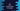# C program to find the surface area of a cube### Write a C program to find the surface area of a cube :

In this example, we will learn how to find the surface area of a cube. A cube has 6 surfaces and the length of its edges are equal length. So, the total surface area is 6 * Surface area of one surface. Surface area of one surface is edge * edge. So, the complete surface area of the cube is (6 * edge * edge). Using C program, we can write it as below :

### C program :

``````#include <stdio.h>
#include <math.h>

//4
float calculateArea(float edge)
{
return 6 * pow(edge, 2);
}

int main()
{
//1
float edge;
float area;

//2
printf("Enter the length of edge of the cube : ");
scanf("%f", &edge);

//3
area = calculateArea(edge);

//5
printf("Surface area of the cube is = %f ", area);
}``````

### Explanation :

The commented numbers above denotes the steps numbers below .

1. Create two floating variables to store the value of length of a edge and area : edge and area.
2. Get the length of the edge from the user and save it in variable edge.
3. Calculate the area and save it in variable area.
4. Function calculateArea(float edge) is used to calculate the surface area of the cube. The calculated area is a floating value.
5. Finally, print out the area of the cube.

### Sample Output :

``````Enter the length of edge of the cube : 3
Surface area of the cube is = 54.000000

Enter the length of edge of the cube : 2.5
Surface area of the cube is = 37.500000

Enter the length of edge of the cube : 14.6
Surface area of the cube is = 1278.960083``````